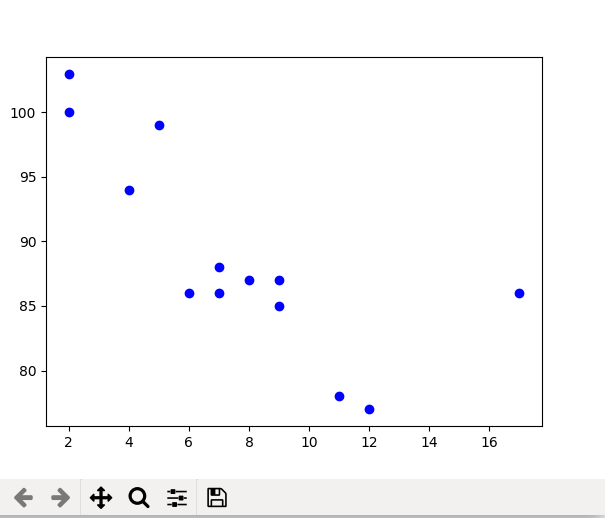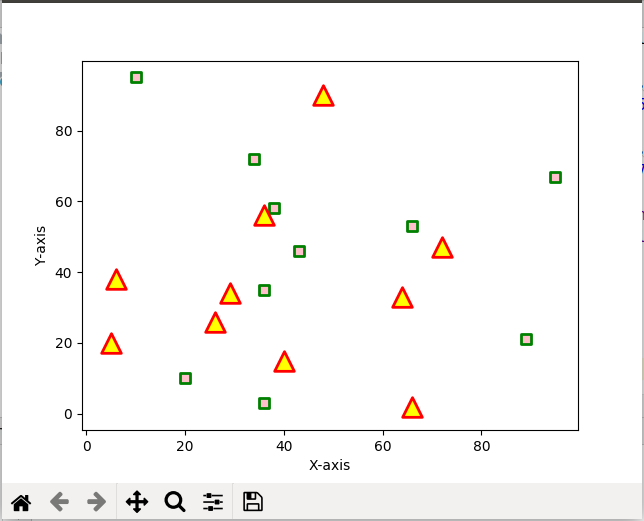# matplotlib.pyplot.scatter() in Python

Matplotlib is a comprehensive library for creating static, animated, and interactive visualizations in Python. It is used for plotting various plots in Python like scatter plot, bar charts, pie charts, line plots, histograms, 3-D plots and many more. We will learn about the scatter plot from the matplotlib library.

## matplotlib.pyplot.scatter()

Scatter plots are used to observe relationship between variables and uses dots to represent the relationship between them. The scatter() method in the matplotlib library is used to draw a scatter plot. Scatter plots are widely used to represent relation among variables and how change in one affects the other.
Syntax
The syntax for scatter() method is given below:

matplotlib.pyplot.scatter(x_axis_data, y_axis_data, s=None, c=None, marker=None, cmap=None, vmin=None, vmax=None, alpha=None, linewidths=None, edgecolors=None)

The scatter() method takes in the following parameters:

• x_axis_data- An array containing x-axis data
• y_axis_data- An array containing y-axis data
• s- marker size (can be scalar or array of size equal to size of x or y)
• c- color of sequence of colors for markers
• marker- marker style
• cmap- cmap name
• linewidths- width of marker border
• edgecolor- marker border color
• alpha- blending value, between 0 (transparent) and 1 (opaque)

Except x_axis_data and y_axis_data all other parameters are optional and their default value is None. Below are the scatter plot examples with various parameters.

Example 1: This is the most basic example of a scatter plot.

 `import` `matplotlib.pyplot as plt ` ` `  ` `  `x ``=``[``5``, ``7``, ``8``, ``7``, ``2``, ``17``, ``2``, ``9``, ` `    ``4``, ``11``, ``12``, ``9``, ``6``]  ` ` `  `y ``=``[``99``, ``86``, ``87``, ``88``, ``100``, ``86``,  ` `    ``103``, ``87``, ``94``, ``78``, ``77``, ``85``, ``86``] ` ` `  `plt.scatter(x, y, c ``=``"blue"``) ` ` `  `# To show the plot ` `plt.show() `

OutputExample 2: Scatter plot with different shape and colour for two datasets.

 `import` `matplotlib.pyplot as plt ` ` `  `# dataset-1 ` `x1 ``=` `[``89``, ``43``, ``36``, ``36``, ``95``, ``10``,  ` `      ``66``, ``34``, ``38``, ``20``] ` ` `  `y1 ``=` `[``21``, ``46``, ``3``, ``35``, ``67``, ``95``,  ` `      ``53``, ``72``, ``58``, ``10``] ` ` `  `# dataset2 ` `x2 ``=` `[``26``, ``29``, ``48``, ``64``, ``6``, ``5``, ` `      ``36``, ``66``, ``72``, ``40``] ` ` `  `y2 ``=` `[``26``, ``34``, ``90``, ``33``, ``38``,  ` `      ``20``, ``56``, ``2``, ``47``, ``15``] ` ` `  `plt.scatter(x1, y1, c ``=``"pink"``,  ` `            ``linewidths ``=` `2``,  ` `            ``marker ``=``"s"``,  ` `            ``edgecolor ``=``"green"``,  ` `            ``s ``=` `50``) ` ` `  `plt.scatter(x2, y2, c ``=``"yellow"``, ` `            ``linewidths ``=` `2``, ` `            ``marker ``=``"^"``,  ` `            ``edgecolor ``=``"red"``,  ` `            ``s ``=` `200``) ` ` `  `plt.xlabel(``"X-axis"``) ` `plt.ylabel(``"Y-axis"``) ` `plt.show() `

OutputMy Personal Notes arrow_drop_upIf you like GeeksforGeeks and would like to contribute, you can also write an article using contribute.geeksforgeeks.org or mail your article to contribute@geeksforgeeks.org. See your article appearing on the GeeksforGeeks main page and help other Geeks.

Please Improve this article if you find anything incorrect by clicking on the "Improve Article" button below.

Article Tags :

Be the First to upvote.

Please write to us at contribute@geeksforgeeks.org to report any issue with the above content.